# Converting decimals to fractions

## Conversion of decimal fractions in the usual

In practice, often use decimals, but when a problem is encountered and common and decimal fractions, you should go for one type of fractions (translate decimal fractions in the ordinary or ordinary in decimal). Not always ordinary fraction can be converted to decimal, so the decimal translate.

When translating decimal fractions in the usual in the numerator of the fraction write the number
what is after the decimal, and the bit unit in the denominator (and so on) contains the same number of zeros as digits after the decimal point in decimal fractions.

### Examples convert the decimal fractions in the usual

Example 1: convert the decimal fraction to normal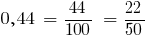If the decimal contains a integer part, convert it into a mixed number and a fractional part of the record before: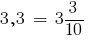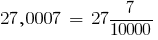## Converting fractions to decimal

Not all fractions can be converted to decimal!

For example, fractions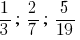cannot be extended to the denominator turned out
10, 100, 1000, etc., Such fractions will be dealt with in high school.

To convert fractions to decimals, you need to:

• to expand on this number so that the denominator was equal to 10, 100, 1000, etc.
• and then the resulting common fraction convert to decimal

### Examples of converting fractions to decimal

Example 2: Turn an ordinary fraction to a decimal: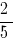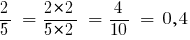Example 3: Translate a common fraction into a decimal: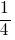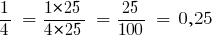In the case of a mixed number, expand the fractional part and the integer part does not change.

Example 4: Turn the mixed number into a decimal: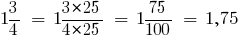Tags:
Chapter:
Versions in other languages:
Share with friends: